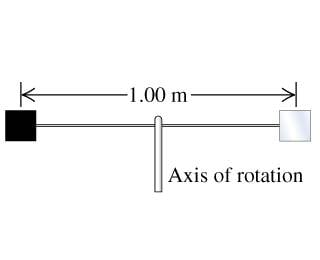# Angular Acceleration due to Light Waves

• JackFlash

## Homework Statement

Two square reflectors, each 1.00 cm on a side and of mass 4.00 g, are located at opposite ends of a thin, extremely light, 1.00-m rod that can rotate without friction and in a vacuum about an axle perpendicular to it through its center (the figure ). These reflectors are small enough to be treated as point masses in moment-of-inertia calculations. Both reflectors are illuminated on one face by a sinusoidal light wave having an electric field of amplitude 1.15 N/C that falls uniformly on both surfaces and always strikes them perpendicular to the plane of their surfaces. One reflector is covered with a perfectly absorbing coating, and the other is covered with a perfectly reflecting coating.## Homework Equations

Near as I can tell:
Emax = cBmax
Prad for Perfect absorbtion = Intensity/c
Prad for Perfect reflection = 2*Intensity/c
Intensity = (Emax * Bmax)/(2μo)
Moment of Inertia for Rod = (mL2)/12
Parallel Axis Theorem: Icm=I + md2
Angular acceleration α = ω2r
Angular frequency ω2 = mgd/Inertia

## The Attempt at a Solution

I calculated Bmax and used 4πx10^-7 for μo to get the Intensity of the light. Considering the light is hitting both cubes, both receive a Radiation Pressure corresponding to whether they absorb the waves or reflect them. I assume the forces on the areas will cancel to some degree, leaving a Radiation Pressure of I/c on the right cube (that one looks like it is supposed to be the reflecting cube). Trying to yield an acceleration by using the equation a = I(A)/mc (since pressure is force/area), where m is the mass of both cubes gives me horribly large and horribly wrong results.

The moment of inertia for a rod is normally (mL2)/12, but the question claims the rod is "extremely light", which I seem to notice usually means the rod has negligable mass. I resort to the Parallel Axis theorem to solve for the moment of inertia of this magic device, which would mean Icm= 2md2, since both cubes are of equal masses and equal distance from the pivot point of the rod. Substituting in for mgd/Inertia to get ω2 and multiplying by r to get α yields incorrect results.

I suppose what I'm asking is whether I'm on the right track (which doesn't seem likely, considering my results being so unrealistic) and what I should consider when facing an equation where "m" is needed, as I'm a bit confused as to whether I should be using the mass of both cubes or the mass of only one.

I calculated Bmax and used 4πx10^-7 for μo to get the Intensity of the light. Considering the light is hitting both cubes, both receive a Radiation Pressure corresponding to whether they absorb the waves or reflect them. I assume the forces on the areas will cancel to some degree, leaving a Radiation Pressure of I/c on the right cube (that one looks like it is supposed to be the reflecting cube).
OK.
Trying to yield an acceleration by using the equation a = I(A)/mc (since pressure is force/area), where m is the mass of both cubes gives me horribly large and horribly wrong results.
Find the force on each square, not the acceleration.

You're on the right track. The force on each square will be quite small. Find the torque exerted.

Alright, so the force on the black square would be (Area)(Intensity)/c and the force on the reflecting square would be twice that. As I mentioned earlier, the apparent (if that is the right word) force would be I/c on the right block.
Torque (τ) is Fd and α is τ/Inertia. Plugging in all I know:
α = (Intensity)(Area)(d)/(c)(.5md^2), where .5md^2 was my moment of inertia. Still wrong. I suspect I have an incorrect moment of inertia, but am confused as to how to calculate it if the rod has a mass that is "extremely light". Using the Parallel Axis theorem gives (2md^2)/4, since both blocks are .5 from the pivot and weigh the same.

Alright, so the force on the black square would be (Area)(Intensity)/c and the force on the reflecting square would be twice that. As I mentioned earlier, the apparent (if that is the right word) force would be I/c on the right block.
OK. (Times area, of course.)
Torque (τ) is Fd and α is τ/Inertia.
Torque would be Fd/2, assuming d is the distance between the masses.
Plugging in all I know:
α = (Intensity)(Area)(d)/(c)(.5md^2), where .5md^2 was my moment of inertia. Still wrong. I suspect I have an incorrect moment of inertia, but am confused as to how to calculate it if the rod has a mass that is "extremely light". Using the Parallel Axis theorem gives (2md^2)/4, since both blocks are .5 from the pivot and weigh the same.
Nothing wrong with your moment of inertia, again assuming that d is the distance between the masses.

I found the correct answer (1.46x10-13) after a bit of trouble (I was calculating Intensity wrong, haha...). I'm going to post a quick run through of my work so that anyone who invariably stumbles upon this problem might see it worked out neatly.

Intensity = Emax2/2μoc = .001754
Prad total is Intensity/c on the silvery square.
F = (Intensity)(Area)/c
Torque τ = Fd = α(Inertia).
(Inertia) = md2 + md2 (for both cubes on the weightless rod)

Values:
d = 1/2m, m = .004kg (force acts on one square), Area = 1x10-4
(Inertia) = m/2

Bring it all together with some fancy substitutions:
α = 2(Intensity)(Area)/2cm = ANS.

Many thanks for the help. If I ever encounter you in real life, I shall give you a burrito.

Angular Acceleration

Hey i am working on this same problem will you help me?